# KSEEB Solutions for Class 9 Maths Chapter 7 Quadrilaterals Ex 7.2

In this chapter, we provide KSEEB SSLC Class 9 Maths Chapter 7 Quadrilaterals Ex 7.2 for English medium students, Which will very helpful for every student in their exams. Students can download the latest KSEEB SSLC Class 9 Maths Chapter 7 Quadrilaterals Ex 7.2 pdf, free KSEEB SSLC Class 9 Maths Chapter 7 Quadrilaterals Ex 7.2 pdf download. Now you will get step by step solution to each question.

## Karnataka Board Class 9 Maths Chapter 7 Quadrilaterals Ex 7.2

Question 1.
ABCD is a quadrilateral in which P, Q, R and S are mid-points of the sides AB, BC, CD and DA. AC is a diagonal. Show that :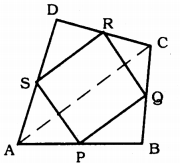(i) SR || AC and SR = 12 AC
(ii) PQ = SR
(iii) PQRS is a parallelogram.
Solution:
Data: ABCD is a quadrilateral in which P, Q, R, and S are mid-points of the sides AB, BC, CD and DA. AC is a diagonal.
To Prove: (i) SR || AC and SR = 12 AC
(ii) PQ = SR
(iii) PQRS is a parallelogram.
Proof: (i) In ∆ADC, S, and R are midpoints of AD and DC sides.
As per mid-point theorem,
SR || AC
and SR = 12 AC.

(ii) In ∆ABC, P and Q are mid-points of AB and BC.
As per mid-point theorem,
PQ || AC
and PQ = 12 AC
But, SR = 12 AC (Proved)
∴ PQ = SR

(iii) PQ = SR (Proved)
SR || AC and PQ || AC
∴ SR || PQ
Opposite sides of a quadrilateral PQRS are equal and parallel, hence PQRS is a parallelogram.

Question 2.
ABCD is a rhombus and P, Q, R, and S are the mid-points of the sides AB, BC, CD, and DA respectively. Show that the quadrilateral PQRS is a rectangle.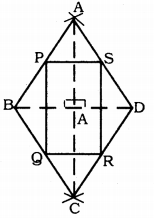Solution:
Data: ABCD is a rhombus and P, Q, R, and S are the mid-points of the sides AB, BC, CD, and DA respectively.
To Prove: PQRS is a rectangle.
Construction: Diagonals AC and BD are drawn.
Proof: To prove PQRS is a rectangle, one of its angles should be the right angle.
In ∆ADC, S and R are the midpoints of AD and DC.
∴ SR || AC
SR = 12 AC (mid-point formula)
In ∆ABC, P and Q are the midpoints AB and BC.
∴ PQ || AC
PQ = 12 AC.
∴ SR || PQ and SR = PQ
∴ PQRS is a parallelogram.
But diagonals of a rhombus bisect at right angles. 90° angle is formed at ’O’.
∴ ∠P = 90°
∴ PQRS is a parallelogram, each of its angles is a right angle.
This is the property of the rectangle.
∴ PQRS is a rectangle.

Question 3.
ABCD is a rectangle and P, Q, R, and S are mid-points of the sides AB, BC, CD, and DA respectively. Show that the quadrilateral PQRS is a rhombus.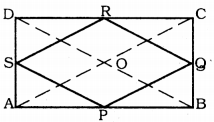Solution:
Data: ABCD is a rectangle and P, Q, R, and S are mid-points of the sides AB, BC, CD, and DA respectively.
To Prove: PQRS is a rhombus.
Construction: Diagonals AC and BD are drawn.
Proof: In ∆ABC, P and Q are the mid-points of AD and BC.
∴ PQ || AC (Mid-point theorem)
PQ = 12 AC ………….. (i)
Similarly, in ∆ADC, S and R are the mid-points of AD and CD.
∴ SR || AC
SR = 12 AC …………… (ii)
Similarly, in ∆ABD,
SP || BD
SP = 12 BD ……………….. (iii)
Similarly, in ∆BCD,
QR || BD
QR = 12 BD ……………… (iv)
From (i), (ii), (iii) and (iv),
PQ = QR = SR = PS and Opposite sides are parallel.
∴ PQRS is a rhombus.

Question 4.
ABCD is a trapezium in which AB || DC, BD is a diagonal and E is the mid-point of AD. A line is drawn through E parallel to AB intersecting BC at F. Show that F is the mid-point of BC.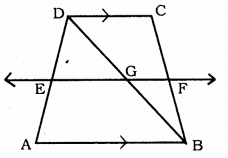Solution:
Data: ABCD is a trapezium in which AB || DC, BD is a diagonal, and E is the mid-point of AD.
A line is drawn through E parallel to AB intersecting BC at F.
To Prove: F is the mid-point of BC.
Proof: Straight line EF cuts at G.
In ∆ABD, E is the mid-point of AD (Data)
EF || AB
EG || AB
∴ G is the mid-point of BD.
∵ Converse of mid-point formula.
DC || AB and EF || AB
⇒ DC || EF
In ∆BDC,
GF || DC
G is the mid-point of BD (proved)
∴ F is the mid-point of BC.

Question 5.
In a parallelogram ABCD, E and F are the mid-points of sides AB and CD respectively. Show that the line segments AF and EC trisect the diagonal BD.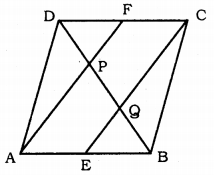Solution:
Data: In a parallelogram ABCD, E and F are the mid-points of sides AB and CD respectively.
To Prove: Line segments AF and EC trisect the diagonal BD.
Proof: ABCD is a parallelogram.
AB || DC and AB = DC
12 AB = 12 DC
AE = CF
and AE || CF (∵ AB || CD)
∴ AE || CF and AE = CF.
∴ AECF is a parallelogram.
∴ AF || EC
In ∆DQC, PF || QC (∵ AF || EC)
∴ P is the mid-point of DQ.
∴ DP = PQ ………….. (i)
In ∆APB, EQ || AP.
But E is the mid-point of AB (Data)
∴ Q is the mid-point of PB.
∴ PQ = QB …………… (ii)
From (i) and (ii),
DP = PQ = QB
∴ AF and EC line segments trisect the diagonal BD.

Question 6.
Show that the line segments joining the mid-points of the opposite sides of a quadrilateral bisect each other.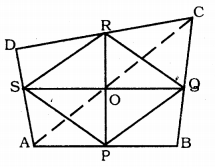Solution:
Data : P, Q, R and S are mid-points of AB, BC, CD and DA respectively in quadrilateral ABCD.
To Prove: PR and SQ line segments bisect mutually.
Construction: Join the diagonal AC.
S and R are the mid-points of AD and DC.
∴ SR || AC
SR = 12 AC ………… (i) (Mid-point Theorem)
Similarly, in ∆ABC,
PQ || AC
PQ = 12 AC …………. (ii)
From (i) and (ii),
SR = PQ and SR || PQ
∴ PQRS is a parallelogram.
PR and SQ are the diagonals of parallelogram PQRS.
∴PR and SQ meet at O’.

Question 7.
ABC is a triangle right angled at C. A line through the mid-point M of hypotenuse AB and parallel to BC intersects AC at D. Show that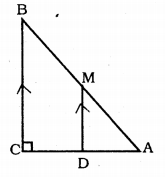(i) D is the mid-point of AC.
(ii) MD ⊥ AC
(iii) CM = MA = 12AB.
Solution:
Data: ABC is a triangle right angled at C. A line through the mid-point M of hypotenuse AB and parallel to BC intersects AC at D.
To Prove: (i) D is the mid-point of AC.
(ii) MD ⊥ AC
(iii) CM = MA = 12 AB.
Proof: (i) In ∆ABC,
M is the mid point of AB and
MB || BC Mid-point of BC (Data)
∴ D is the mid-point of AC (Mid-point formula)

(ii) MD || BC
∠BCD + ∠MDC = 180° (Sum of interior angles)
90 + ∠MDC = 180°
∠MDC = 180 – 90
∠MDC = 90°
∴ MD ⊥ AC

(iii) In this fig. CM is joined.
M is the mid-point of AB.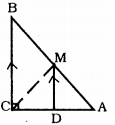∴ MA = 12 AB ……….. (i)
Now, in ∆AMD and ∆CMD.
∠MDC = ∠MDA = 90°
MD is common.
∴∆AMD ≅ ∆CMD (SAS Postulate)
∴ CM = MA …………… (ii)
Comparing (i) and (ii),
CM = MA = 12 AB.

All Chapter KSEEB Solutions For Class 9 Maths

—————————————————————————–

All Subject KSEEB Solutions For Class 9

*************************************************

I think you got complete solutions for this chapter. If You have any queries regarding this chapter, please comment on the below section our subject teacher will answer you. We tried our best to give complete solutions so you got good marks in your exam.

If these solutions have helped you, you can also share kseebsolutionsfor.com to your friends.

Best of Luck!!